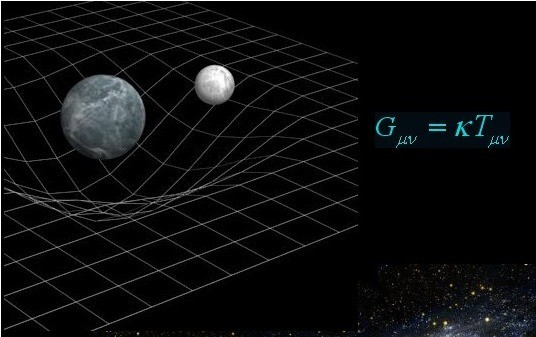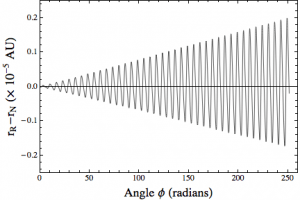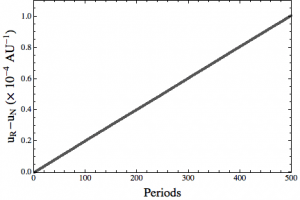# Secular and Periodic

In Relativity by Brian Koberlein4 Comments

More general relativity today. This time a bit on how to calculate the perihelion advance of Mercury in general relativity. When you derive the central force equation for relativistic gravity you find there is an extra term not seen in Newton’s gravity. The extra term is small, but enough to make Mercury’s orbit (any orbit really, but we typically use Mercury as an example) deviate slightly from an ellipse. Since the deviation is small, you can make some broad approximations, get an approximate solution for Mercury’s orbit, then determine the perihelion advance for one orbit.That’s all fine and good if all you want is an approximate solution, but what if you really want to grind through things and find an exact answer. It turns out you can’t get a general analytic solution for the GR case, but you can solve it computationally. Do this in Mathematica and you can get an interpolating function. Okay, so get your interpolating function and figure the shift for one orbit. Problem solved, right?Well, not so fast. Just doing it for one orbit is not very accurate, so its better to calculate the solution over several hundred orbits. But then you run into a subtlety of relativistic orbits. It turns out the perihelion advance is only part of what is going on. There are actually two types of non-Newtonian behaviors. One is a secular deviation (the perihelion advance), and the other is a periodic deviation.

You can see both of them in the first figure above. I’ve plotted the deviation between Newton’s orbit and Einstein’s, and what you can see is there is a kind of periodic motion with increasing amplitude. If we just take an average of this difference, everything washes out. What we want to find is how the amplitude increases over time. So we have to do a fit to the average amplitude increase. The result is seen in the second figure, which gives the steady advance of the perihelion.

## Comments

1.I think I’ll just take your word on that one.

2.A link with some details would be nice. I did the calculation of the secular deviation when I took classical mechanics as a freshman – I still remember really sweating through that exercise in Goldstein’s Classical Mechanics. But I’ve never even heard of the periodic deviation!

1.Author

It’s actually in my book, “Astrophysics Through Computation”, in chapter 5 on relativity.